# Selina Solutions Concise Maths Class 10 Chapter 17 Circles Exercise 17(B)

This exercise deals with problems based on important theorems and results of circles. Students having any doubts regarding solving problems of ICSE Class 10 Mathematics can refer to the Selina Solutions for Class 10 Math. It contains solutions, which are as per the latest ICSE examination patterns. Further, the solutions of this exercise are available in the Concise Selina Solutions for Class 10 Maths Chapter 17 Circles Exercise 17(B) PDF, link given below.

## Selina Solutions Concise Maths Class 10 Chapter 17 Circles Exercise 17(B) Download PDF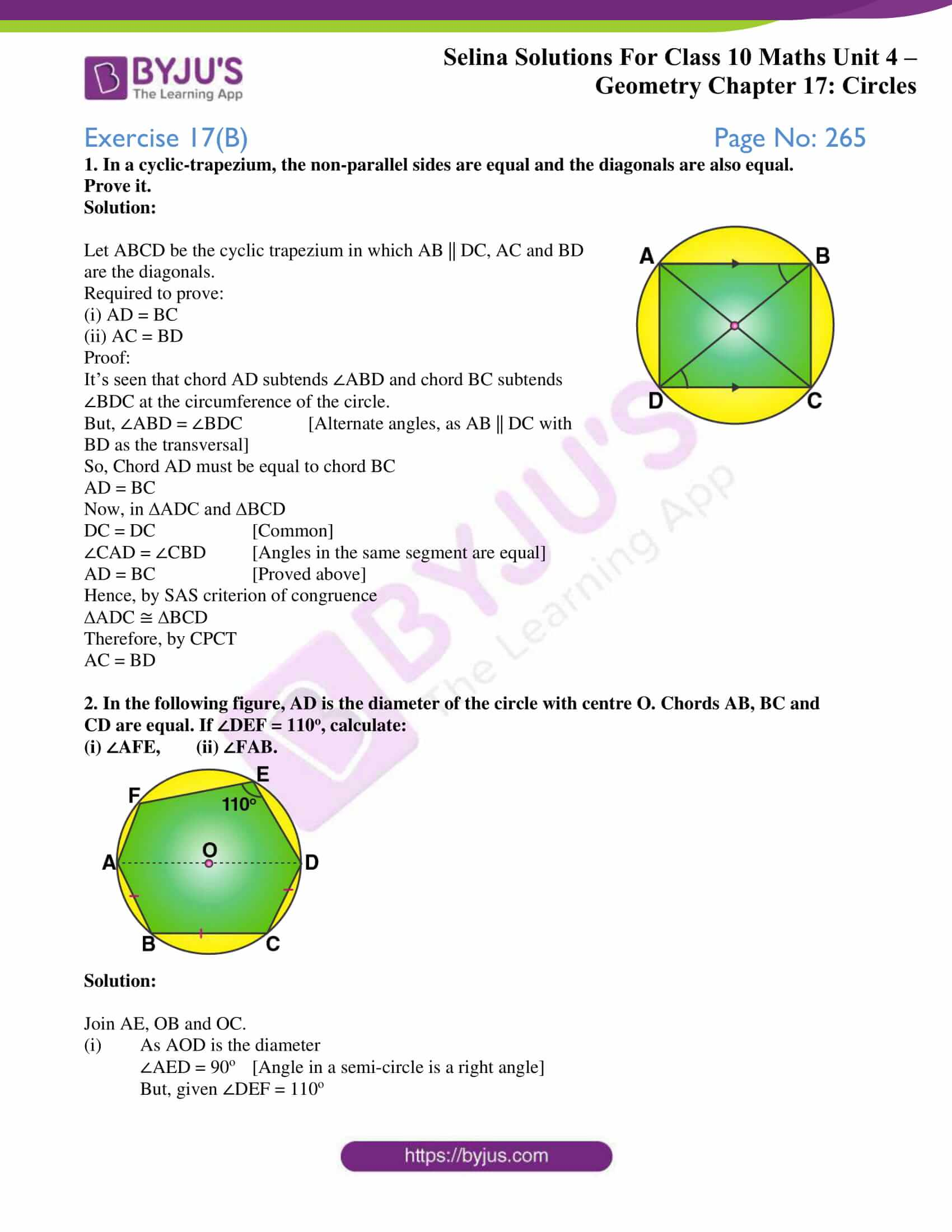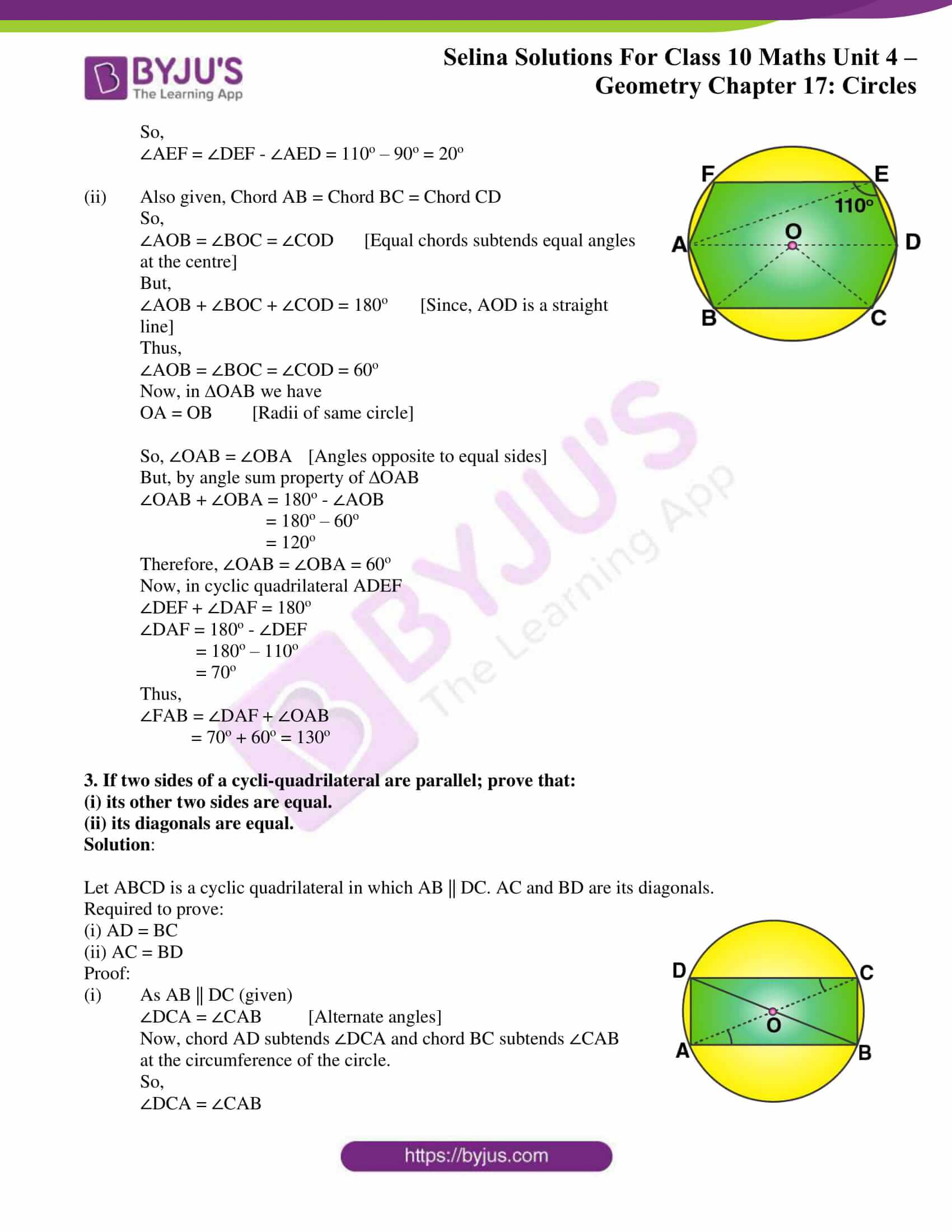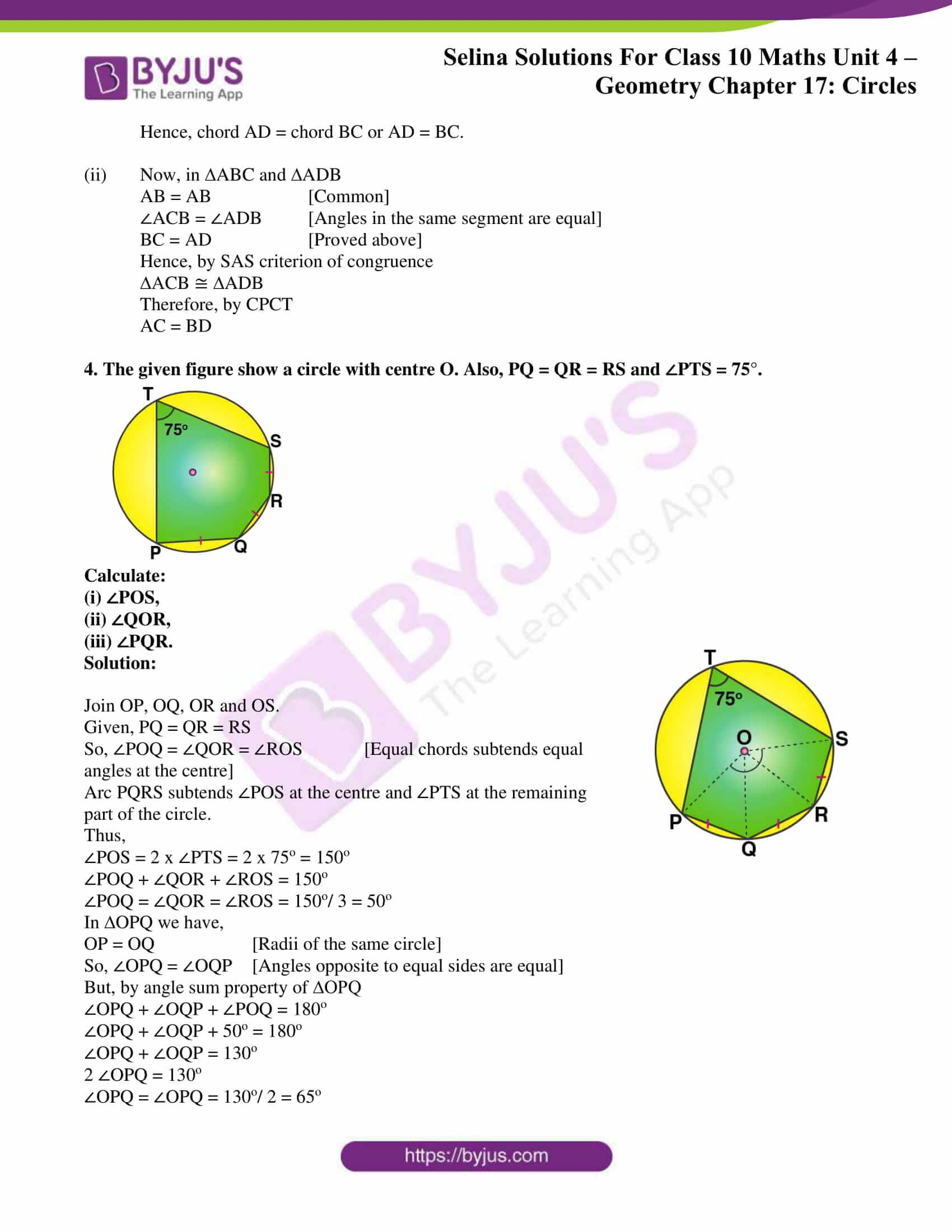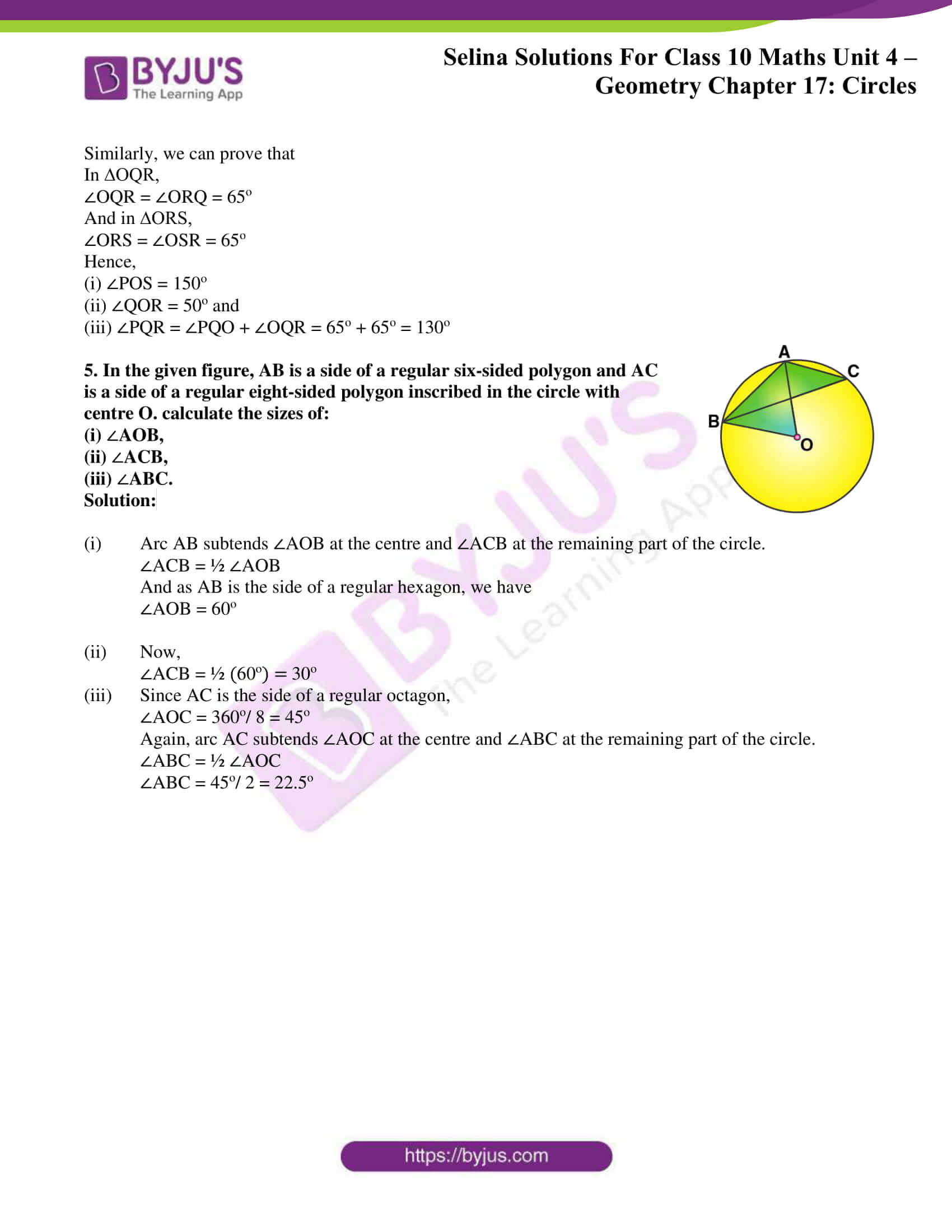### Access Selina Solutions Concise Maths Class 10 Chapter 17 Circles Exercise 17(B)

1. In a cyclic-trapezium, the non-parallel sides are equal and the diagonals are also equal.

Prove it.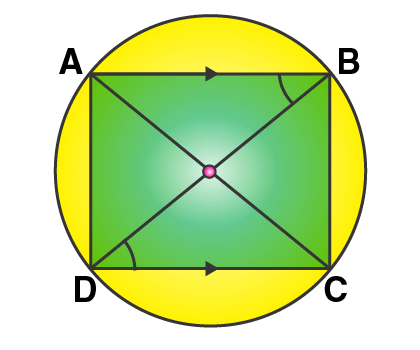Solution:

Let ABCD be the cyclic trapezium in which AB || DC, AC and BD are the diagonals.

Required to prove:

(ii) AC = BD

Proof:

It’s seen that chord AD subtends ∠ABD and chord BC subtends ∠BDC at the circumference of the circle.

But, ∠ABD = ∠BDC [Alternate angles, as AB || DC with BD as the transversal]

So, Chord AD must be equal to chord BC

DC = DC [Common]

∠CAD = ∠CBD [Angles in the same segment are equal]

Hence, by SAS criterion of congruence

Therefore, by CPCT

AC = BD

2. In the following figure, AD is the diameter of the circle with centre O. Chords AB, BC and CD are equal. If ∠DEF = 110o, calculate:

(i) ∠AFE, (ii) ∠FAB.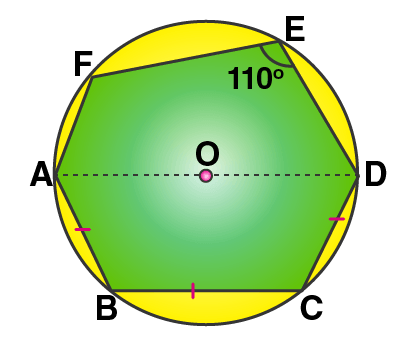Solution:

Join AE, OB and OC.

(i) As AOD is the diameter

∠AED = 90o [Angle in a semi-circle is a right angle]

But, given ∠DEF = 110o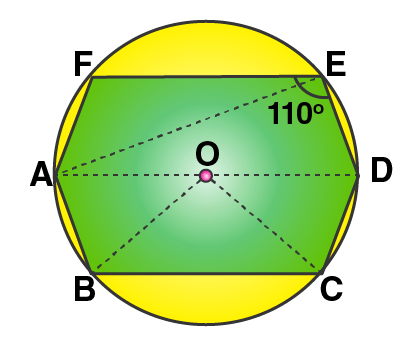So,

∠AEF = ∠DEF – ∠AED = 110o – 90o = 20o

(ii) Also given, Chord AB = Chord BC = Chord CD

So,

∠AOB = ∠BOC = ∠COD [Equal chords subtends equal angles at the centre]

But,

∠AOB + ∠BOC + ∠COD = 180o [Since, AOD is a straight line]

Thus,

∠AOB = ∠BOC = ∠COD = 60o

Now, in ∆OAB we have

OA = OB [Radii of same circle]

So, ∠OAB = ∠OBA [Angles opposite to equal sides]

But, by angle sum property of ∆OAB

∠OAB + ∠OBA = 180o – ∠AOB

= 180o – 60o

= 120o

Therefore, ∠OAB = ∠OBA = 60o

∠DEF + ∠DAF = 180o

∠DAF = 180o – ∠DEF

= 180o – 110o

= 70o

Thus,

∠FAB = ∠DAF + ∠OAB

= 70o + 60o = 130o

3. If two sides of a cycli-quadrilateral are parallel; prove that:

(i) its other two sides are equal.

(ii) its diagonals are equal.

Solution:

Let ABCD is a cyclic quadrilateral in which AB || DC. AC and BD are its diagonals.

Required to prove: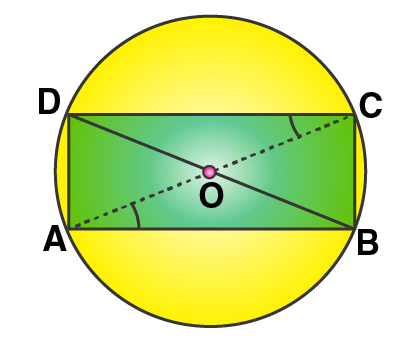(i) AD = BC

(ii) AC = BD

Proof:

(i) As AB || DC (given)

∠DCA = ∠CAB [Alternate angles]

Now, chord AD subtends ∠DCA and chord BC subtends ∠CAB at the circumference of the circle.

So,

∠DCA = ∠CAB

(ii) Now, in ∆ABC and ∆ADB

AB = AB [Common]

∠ACB = ∠ADB [Angles in the same segment are equal]

Hence, by SAS criterion of congruence

Therefore, by CPCT

AC = BD

4. The given figure show a circle with centre O. Also, PQ = QR = RS and ∠PTS = 75°.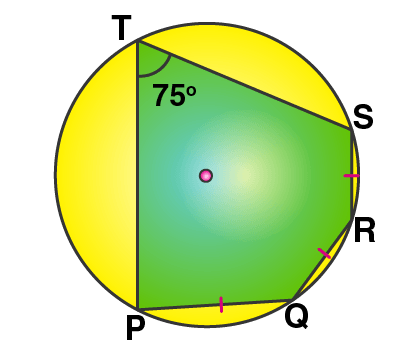Calculate:

(i) ∠POS,

(ii) ∠QOR,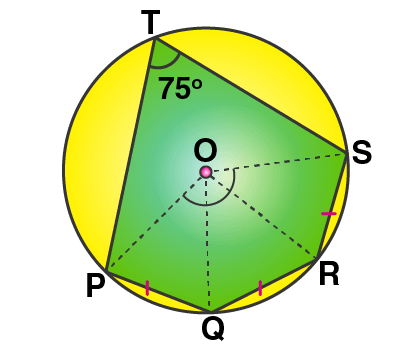(iii) ∠PQR.

Solution:

Join OP, OQ, OR and OS.

Given, PQ = QR = RS

So, ∠POQ = ∠QOR = ∠ROS [Equal chords subtends equal angles at the centre]

Arc PQRS subtends ∠POS at the centre and ∠PTS at the remaining part of the circle.

Thus,

∠POS = 2 x ∠PTS = 2 x 75o = 150o

∠POQ + ∠QOR + ∠ROS = 150o

∠POQ = ∠QOR = ∠ROS = 150o/ 3 = 50o

In ∆OPQ we have,

OP = OQ [Radii of the same circle]

So, ∠OPQ = ∠OQP [Angles opposite to equal sides are equal]

But, by angle sum property of ∆OPQ

∠OPQ + ∠OQP + ∠POQ = 180o

∠OPQ + ∠OQP + 50o = 180o

∠OPQ + ∠OQP = 130o

2 ∠OPQ = 130o

∠OPQ = ∠OPQ = 130o/ 2 = 65o

Similarly, we can prove that

In ∆OQR,

∠OQR = ∠ORQ = 65o

And in ∆ORS,

∠ORS = ∠OSR = 65o

Hence,

(i) ∠POS = 150o

(ii) ∠QOR = 50o and

(iii) ∠PQR = ∠PQO + ∠OQR = 65o + 65o = 130o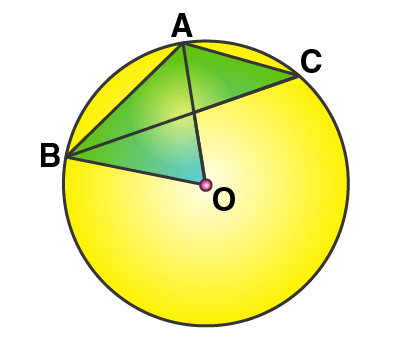5. In the given figure, AB is a side of a regular six-sided polygon and AC is a side of a regular eight-sided polygon inscribed in the circle with centre O. calculate the sizes of:

(i) AOB,

(ii) ACB,

(iii) ABC.

Solution:

(i) Arc AB subtends ∠AOB at the centre and ∠ACB at the remaining part of the circle.

∠ACB = ½ ∠AOB

And as AB is the side of a regular hexagon, we have

∠AOB = 60o

(ii) Now,

∠ACB = ½ (60o) = 30o

(iii) Since AC is the side of a regular octagon,

∠AOC = 360o/ 8 = 45o

Again, arc AC subtends ∠AOC at the centre and ∠ABC at the remaining part of the circle.

∠ABC = ½ ∠AOC

∠ABC = 45o/ 2 = 22.5o